# Finding the center of projection of a perspective projection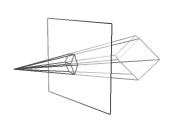A perspective projection creates 2D images of 3D objects by projecting lines from a center of projection through a image plane until they meet the objects. The intersections of the projection lines with the image plane form the 2D images of the 3D objects.

The distance between the center of projection and the image plane affects how flat or deep the 2D images appear. It also controls how much of the 3D world appears in the image. If you have a perspective image and know the the distance to the center of projection that was used to create it, you can calculate information about the 3D objects.

This page describes a method to find the distance between the center of projection and the image plane in a perspective projection.

## Restrictions and assumptions

This method works with true perspective projections that transform straight world lines into straight image lines. Be aware that real cameras don't create mathematically perfect perspective projections and can render straight world lines as curved image lines. This might reduce the accuracy of this method. 3D video game screenshots or other 3D computer graphics are true perspective projections and this method should work well with them.

This method assumes that the center of projection is directly in front of the center point of the image plane. This is usually the case. However, you need to make sure the image hasn't been cropped or you won't be able to find the original center point of the image.

This method relies on finding two groups of parallel lines that form right angles with each other and that aren't parallel to the image plane. These are easiest to find if the image contains the corner of a box or building, or a floor tiled with rectangles viewed from a corner.

## Steps of the method

Here's a screenshot from Sonic Adventure I'll use to illustrate the method.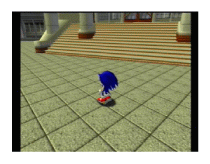1. Find a floor tiled with rectangles, or the corner of a box or building. Identify a group of parallel lines on the edges of the tiles, box, or building. Draw lines that extend the images of the parallel lines until they intersect. The intersection point is the vanishing point for this group of parallel lines.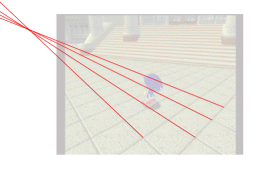2. Identify another group of parallel lines on the edges of the tiles, box, or building that make right angles with the first group of parallel lines. Draw lines to extend the images of these parallel lines until they intersect. This intersection point is the vanishing point for this second group of parallel lines.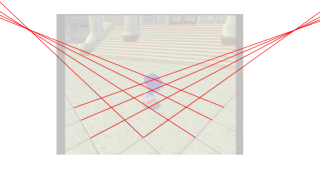3. Draw a line between the two vanishing points. Find the midpoint of this line. Draw a circle centered on the midpoint and with a radius to both of the vanishing points.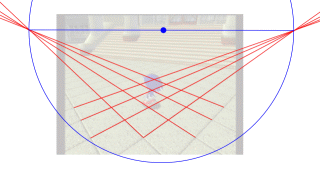This circle represents the intersection of a sphere with the image plane. Imagine the image plane cutting a sphere in half, and this circle as the equator of that sphere.

4. Mark the center point of the image plane. Now draw a chord that passes through the center point of the image plane and stops on either edge of the circle. I made the chord horizontal, but it can be any chord that passes through the center point of the image plane.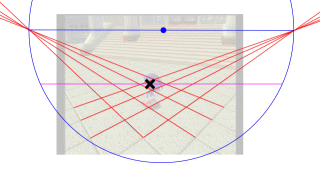5. Find the midpoint of the chord. Draw a semicircle centered on this midpoint and with a radius to both ends of the chord.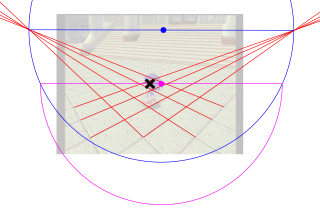Imagine folding this semicircle upwards 90 degrees and you can see it represents a cross section of the sphere.

6. Draw a line starting from the center point of the image plane, perpendicular to the chord, until it meets the semicircle.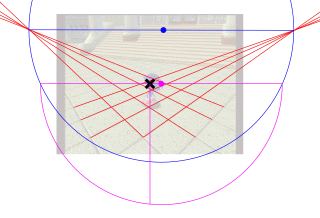The distance of this line represents the distance from the center point of the image plane to the center of projection. If you imagine folding the semicircle upwards 90 degrees, then the line would end at the exact same location as the center of projection.

In this example, I imported the screenshot into Inkscape and carefully constructed the lines and circles on top of it. The screenshot was 320 pixels wide and 240 pixels high, and the distance to the center of projection was 207.2 pixels.

## How it works

The method uses the following facts and assumption: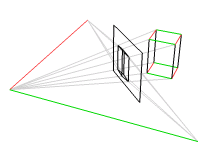Fact 1: Any group of parallel lines that isn't parallel to the image plane will have images in the image plane that converge at a vanishing point. The line between the center of projection and the vanishing point is also parallel to the other lines.1

For example, in the diagram on the right, the red lines are parallel and the green lines are parallel.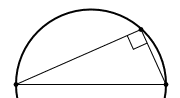Fact 2: If you have two points a and b, and you're trying to find a third point c that forms a right angle with a and b, then c must be somewhere on a circle whose diameter is the line segment between a and b. (See Thales' theorem for a proof.) If a, b, and c are three-dimensional points, then c must be somewhere on a sphere whose diameter is the line segment between a and b.

Assumption 3: The center of projection is directly in front of the center point of the image plane.

The method uses the images of right angled objects to find the vanishing points of the lines that form right angles. Because of fact 1, the angle between the center of projection and the vanishing points is also a right angle. Because of fact 2, the center of projection must be somewhere on a sphere whose diameter is the line segment between the vanishing points. And using assumption 3, the method effectively draws a line from the center point of the image plane, perpendicular to the image plane, until it meets the sphere. The center of projection is located where that line meets the sphere.

## Alternate method

Find the two vanishing points as described above. Then imagine the image plane has a coordinate system with the origin at the center of the image.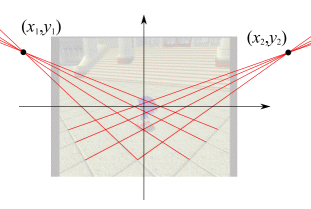Find the coordinates of the two vanishing points (x1, y1) and (x2, y2). Then calculate the distance d between the center of the image plane and the center of projection as follows.

d = √x1x2y1y2

The example screenshot is 320 pixels wide and 240 pixels high. The vanishing point coordinates are

x1 = -207.2 pixels
y1 = 92.4 pixels
x2 = 247.3 pixels
y2 = 92.4 pixels

Using this method, the distance to the center of projection is d = 206.6 pixels.

## How the alternate method works

The formula for d is derived by using the distance formula and the Pythagorean theorem.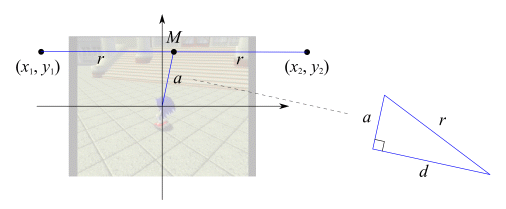The two vanishing points are at (x1, y1) and (x2, y2).
The radius r of the sphere is the half the distance between the vanishing points

r = (1/2) √ (x2x1)2 + (y2y1)2

The center of the image is at (0, 0).
The midpoint M between the vanishing points is at ( (x1+x2)/2, (y1+y2)/2 ).
The distance a from the center of the image to M is

a = √ ((x1+x2)/2)2 + ((y1+y2)/2)2

Imagine a right triangle pointing outward from the center of the image, with the right angle on top of the center of the image, with one leg along a, and with a hypotenuse of distance r. Then the other leg is d, the distance to the center of projection. Using the Pythagorean theorem,

a2 + d2 = r2

or

d = √ r2a2

Plugging in r and a and simplifying yields

d = √x1x2y1y2

## Angle of view

The angle of view (or field of view) is an angle the center of projection makes with the edges of the image plane. Different people and systems use different edges of the image plane to define the angle. It can be the angle from the center of projection to the left and right of the image plane, or to the top and bottom of the image plane, or to opposite diagonal corners of the image plane. Or it can be the angle from the center of projection to one edge of the image plane (left, top, or corner) and to the center of the image plane.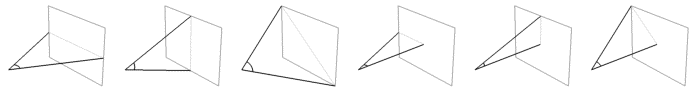The distance between the center of projection and the image plane and the angle of view are alternative ways to specify the location of the center of projection. If you know one of these values, you can use trigonometry to find the other.

For the example screenshot, here are the various dimensions:

 width w = 320 pixels height h = 240 pixels diagonal s = √w² + h² = 400 pixels distance to center of projection d = 207.2 pixels

Here are the different ways to calculate the angle of view:

 left to right 2 tan-1 ( w / 2d ) = 75.4° top to bottom 2 tan-1 ( h / 2d ) = 60.2° corner to corner 2 tan-1 ( s / 2d ) = 88.0°
 left to center tan-1 ( w / 2d ) = 37.7° top to center tan-1 ( h / 2d ) = 30.1° corner to center tan-1 ( s / 2d ) = 44.0°

Thales' theorem from Wikipedia
en.wikipedia.org/wiki/Thales%27_theorem
The theorem and proof that any point on a circle makes a right angle with a diameter of the circle.

Here are some interesting pages I read while researching perspective projection.

Lessons in Mathematics and Art
php.indiana.edu/~mathart/viewpoints/lessons/

These PDF file lessons describe various perspective mathematics.

Lesson 3 describes methods for finding the center of projection from a one-point perspective image, provided you can find the image of a square, or if you know the dimensions of a rectangle in the image.

The "Perspective Super-Problems" hints at a method for finding the center of projection of a two-point perspective image which is similar to the method on this page. The method assumes the center of projection is centered right-to-left, but not top-to-bottom. Also, in two-point perspective, the center of projection is directly in front of the horizon (the line between the vanishing points), so only one semicircle needs to be drawn.

The "Perspective Super-Problems" also hints at a method for finding the center of projection of a three-point perspective image using three spheres.

Perceiving The Window in Order To See The World (page 1)
from The Arrow in the Eye: The Psychology of Perspective and Renaissance Art
www.webexhibits.org/arrowintheeye/perceiving1.html

The box and image in the middle of this page describe a general method for finding the center of projection when it isn't directly in front of the center point of the image plane. This method involves finding three groups of parallel lines that are at right angles with each other, using their vanishing points to make three spheres, and constructing the intersection of the spheres which is the center of projection.

Determination of the correct eye position for viewing perspective images of 3D scenes [PDF]
www.gvu.gatech.edu/~jarek/papers/Perspective.pdf

This PDF file describes another general method for finding the center of projection by making a "vanishing point triangle", finding its orthocenter, and calculating the distance to the center of projection from those.

Elements of perspective
www.handprint.com/HP/WCL/tech10.html

A very comprehensive tutorial on using perspective in art. The section Where is the center of projection? www.handprint.com/HP/WCL/perspect3.html#projection mentions the same sorts of methods for finding the center of projection.